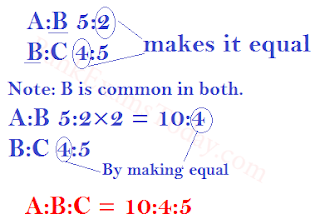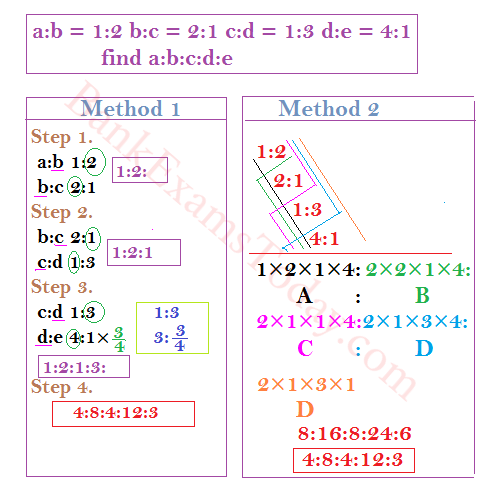Gurpurab Offer - Use Code GURPURAB2023

# Ratio and Proportion Methods with Shortcut Tricks

1. Ratio - Meaning and  Example
2. Proportion - Meaning and Example

## # Ratio

It estimates one quantity is how much times of the other and vice versa but both the quantities should be of same type.

Example 1.  Let's say Ram and Sham have Rs. 20 and 40 respectively .Then their ratio of money is 2:4(a:b). Here First term is called antecedent like a and Second term called consequent like b.It means Ram has 2 times as much money as Sham and also Sham has 4 times as much money as Ram.
Now Assume Ram and Sham have Rs. 20 and \$ 40 respectively. Now First Check whether these two quantity of same type.No! Both the quantities are not the same type because the one quantity is in rupees and the other in dollar.So we cannot able to define it's ratio.

### Some Basic Questions With Explanation

#### If a:b = 5:2 and b:c = 4:5 then a:b:c = ?.#### If a:b = 1:2 b:c = 2:1 c:d = 1:3 d:e = 4:1 then a:b:c:d:e = ?## # Proportion

If Two quantity having the value in same ratio called proportion. A proportion is an expression which states that two ratios are equal.
Let say we have two cane.1st cane has water and syrup in ratio 50:200 and the other has in ratio 25:100 respectively.Here In 1st cane 50 is 1/4 of 200 and also 25 is 1/4th of 100 so both ratios are equal.hence we can say that both are proportional to each other.
Let a:b = 3:12 and b:c = 5:20 because they are in proportion we can say that
a:b = b:c or x:y :: y:z
product of middle term or means = product of extremes#### Find third proportional to 3,12,20 respectively?

Let third proportion is x.
a:b :: b:c or 3:12 :: x:20
x = 3×20/12
x = 5

#### Find fourth proportional to 3,12,5 respectively?

Let fourth proportion is x.
so  a:b :: b:c or 3:12::5:x
x = 12×5/3 [ a:b = b:c ]
x = 20

#### Find Mean Proportional to a number 3 and 75 ?

Let mean proportion is x.
a:b :: b:c  or 3:x :: x:75
(x)2 = 3×75
(x)2 = 225
x = 15

## Important Questions:

### 1. Coins and Values:

In this type of question , you would have asked to find out either no. of coins or values.While calculating you should remember that the ratio of values comes first and then the ratio of no. of coins when it is not given in the question.

#### A bag contains an equal number of one rupee, 50 paise and 25 paise coins respectively. If the total value is Rs. 35, how many coins of each type are there?

Sol:
1 Rs. = 100 paise
Step 1. Consists of coins 100 paise,50paise and 25 paise
so 100:50:25
Step 2. 100:50:25 or 4:2:1
Total value of one rupee coins = 4/7×35 = 20
Total value of fifty paise coins = 4/7×35 = 10
Total value of twenty-five paise coins = 4/7×35 = 5
Step 3.
No. of one rupees coins = 20×1 = 20
No. of fifty paise coins = 10×2 = 20
No. of twenty-five paise coins = 5×4 = 20
Trick :
No. of each type of coin = Total amount/Sum of values of each coin
No. of each type of coin = 35/1+0.5+0.25 => 20 coins of each type

#### In a bag, 25 paise, 10 paise and 5 paise coins are in the ratio of  1:2:3. If their total value is Rs. 30, then the number of 5 paise coins is ?

Sol: coins are in the ratio 1:2:3
values are in the ratio : 1/4:2/10:5/20 or 5:4:3
No. of coins of 5 paise = Toal values of 5 paise coins × No. of coins of 5 paise in 100 paise
Total values of 5 paise coins = 3/12×30 => 15 /2 or Rs. 7.50
No. of coins of 5 paise = 15/2×20=150 or 7.5×20 = 150

#### 465 coins consist of rupee, 50 paise and 25 paise coins. Their values are in the ratio 5:3:1. Find the number of each coin?

Sol:
Values are in the ratio = 5:3:1
Ratio of no. of coins = 5×1:3×2:1×4
5:6:4
the no. of one-rupee coins = 5/15×465 = 155
the no. of fifty-paise coins = 6/15×465 = 186
the no. of one-rupee coins = 4/15×465 = 124

### 2. Income and Expenditure :

In this type of questions ,either income or expenditure or their ratio will ask .

#### The income of A and B is in the ratio 3:2 and expenses are in the ratio 5:3. If both save Rs. 200, then the income of A is ?

Sol:Sol:

### 4. Mixture of Content :

#### The content of two vessels containing water and milk are in the ratio 1:2 and 2:5 are mixed in the ratio 1:4. The resulting mixture will have water and milk in the ratio?

Sol: Lets understand the question.
The Question says there are two vessels A and B.In vessels A , there is a water and milk in different ratio which is 1/3 and 2/3  water and milk respectively(same apply for vessels B).Here in 1/3 , the denominator or lower value (3) implies 3 equal part of content and the numerator or upper value (1) implies 1 part taken to form the content.This is something about fraction.Now lets move to the question.
Simply there are two vessels A and B  and we need to make an another mixture from these two vessels by taking 1/5th part from vessels A and 4/5th part from vessels B.